A proton of mass $1.67×{10}^{-27}kg$ and charge $1.6×{10}^{-19}C$ is projected with a speed of $2×{10}^{6}m/s$ at an angle of $60°$ to the X-axis. If a uniform magnetic field of 0.104 Tesla is applied along Y-axis, the path of the proton is:

1. A circle of radius = 0.2 m and time period $\mathrm{\pi }×{10}^{-7}\mathrm{s}$

2. A circle of radius = 0.1 m and time period $2\mathrm{\pi }×{10}^{-7}\mathrm{s}$

3. A helix of radius = 0.1 m and time period $2\mathrm{\pi }×{10}^{-7}\mathrm{s}$

4. A helix of radius = 0.2 m and time period $4\mathrm{\pi }×{10}^{-7}\mathrm{s}$

Concept Questions :-

Lorentz force
High Yielding Test Series + Question Bank - NEET 2020

Difficulty Level:

The magnetic field at the centre of a circular coil of radius r is $\mathrm{\pi }$ times that due to a long straight wire at a distance r from it, for equal currents. Figure here shows three cases : in all cases the circular part has radius r and straight ones are infinitely long. For same current the B field at the centre P in cases 1, 2, 3 have the ratio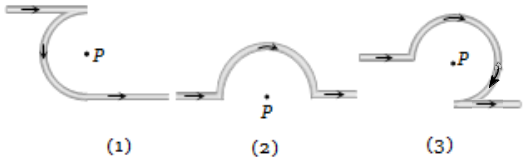(a)

(b) $\left(-\frac{\mathrm{\pi }}{2}+1\right)\mathbf{:}\left(\frac{\mathrm{\pi }}{2}+1\right)\mathbf{:}\left(\frac{3\mathrm{\pi }}{4}+\frac{1}{2}\right)$

(c) $-\frac{\mathrm{\pi }}{2}\mathbf{:}\frac{\mathrm{\pi }}{2}\mathbf{:}3\frac{\mathrm{\pi }}{4}$

(d) $\left(-\frac{\mathrm{\pi }}{2}-1\right)\mathbf{:}\left(\frac{\mathrm{\pi }}{2}-\frac{1}{4}\right)\mathbf{:}\left(\frac{3\mathrm{\pi }}{4}+\frac{1}{2}\right)$

Concept Questions :-

Magnetic field due to various cases
High Yielding Test Series + Question Bank - NEET 2020

Difficulty Level:

A proton and a deuteron both having the same kinetic energy, enter perpendicularly into a uniform magnetic field B. For the motion of proton and deuteron on a circular path of radius ${R}_{p}$and ${R}_{d}$ respectively, the correct statement is:

(a) ${R}_{d}=\sqrt{2}{R}_{p}$            (b) ${R}_{d}={R}_{p}/\sqrt{2}$

(c) ${R}_{d}={R}_{p}$                  (d) ${R}_{d}=2{R}_{p}$

Concept Questions :-

Lorentz force
High Yielding Test Series + Question Bank - NEET 2020

Difficulty Level:

Two straight long conductors AOB and COD are perpendicular to each other and carry currents ${\mathrm{i}}_{1}$ and ${\mathrm{i}}_{2}$ . The magnitude of the magnetic induction at a point P at a distance a from the point O in a direction perpendicular to the plane ACBD is:

1. $\frac{{\mathrm{\mu }}_{0}}{2\mathrm{\pi a}}\left({\mathrm{i}}_{1}+{\mathrm{i}}_{2}\right)$                    2. $\frac{{\mathrm{\mu }}_{0}}{2\mathrm{\pi a}}\left({\mathrm{i}}_{1}-{\mathrm{i}}_{2}\right)$

3. $\frac{{\mathrm{\mu }}_{0}}{2\mathrm{\pi a}}{\left({\mathrm{i}}_{1}^{2}+{\mathrm{i}}_{2}^{2}\right)}^{1/2}$                4. $\frac{{\mathrm{\mu }}_{0}}{2\mathrm{\pi a}}\frac{{\mathrm{i}}_{1}{\mathrm{i}}_{2}}{\left({\mathrm{i}}_{1}+{\mathrm{i}}_{2}\right)}$

Concept Questions :-

Magnetic field due to various cases
High Yielding Test Series + Question Bank - NEET 2020

Difficulty Level:

The charge on a particle Y is double the charge on particle X. These two particles X and Y after being accelerated through the same potential difference enter a region of the uniform magnetic field and describe circular paths of radii ${R}_{1}$ and ${R}_{2}$ respectively. The ratio of the mass of X to that of Y is:

(a) ${\left(\frac{2{R}_{1}}{{R}_{2}}\right)}^{2}$                      (b) ${\left(\frac{{R}_{1}}{2{R}_{2}}\right)}^{2}$

(c)  $\frac{{R}_{1}^{2}}{2{R}_{2}^{2}}$                           (d) $\frac{2{R}_{1}}{{R}_{2}}$

Concept Questions :-

Lorentz force
High Yielding Test Series + Question Bank - NEET 2020

Difficulty Level:

In the given figure, the electron enters into the magnetic field. It deflects in ...... direction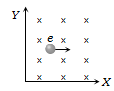(a) + ve X direction

(b) – ve X direction

(c) + ve Y direction

(d) – ve Y direction

Concept Questions :-

Lorentz force
High Yielding Test Series + Question Bank - NEET 2020

Difficulty Level:

A cell is connected between the points A and C of a circular conductor ABCD of centre O with angle AOC = $60°$ . If ${\mathrm{B}}_{1}$ and ${\mathrm{B}}_{2}$ are the magnitudes of the magnetic fields at O due to the currents in ABC and ADC respectively, the ratio $\frac{{\mathrm{B}}_{1}}{{\mathrm{B}}_{2}}$ is: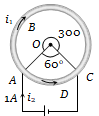1. 0.2

2. 6

3. 1

4. 5

Concept Questions :-

Magnetic field due to various cases
High Yielding Test Series + Question Bank - NEET 2020

Difficulty Level:

An electron, a proton, a deuteron and an alpha particle, each having the same speed are in a region of constant magnetic field perpendicular to the direction of the velocities of the particles. The radius of the circular orbits of these particles are respectively ${R}_{e}$, ${R}_{p}$${R}_{d}$ and ${R}_{\alpha }$. It follows that

(a) ${R}_{e}={R}_{p}$                (b) ${R}_{p}={R}_{d}$

(c) ${R}_{d}={R}_{\alpha }$                 (d) ${R}_{p}={R}_{\alpha }$

Concept Questions :-

Lorentz force
High Yielding Test Series + Question Bank - NEET 2020

Difficulty Level:

An infinitely long conductor PQR is bent to form a right angle as shown. A current I flows through PQR. The magnetic field due to this current at the point M is H1. Now another infinitely long straight conductor QS is connected at Q so that the current is I/2 in QR as well as in QS, The current in PQ remaining unchanged. The magnetic field at M is now ${H}_{\mathit{2}}$The ratio ${H}_{\mathit{1}}\mathit{/}{H}_{\mathit{2}}$ is given by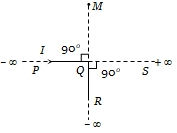(a) $\frac{1}{2}$

(b)  1

(c)  $\frac{2}{3}$

(d)  2

Concept Questions :-

Magnetic field due to various cases
High Yielding Test Series + Question Bank - NEET 2020

Difficulty Level:

A very long straight wire carries a current I. At the instant when a charge $+\underset{~}{O}$ at point P has velocity $\stackrel{\to }{v}$ , as shown, the force on the charge is: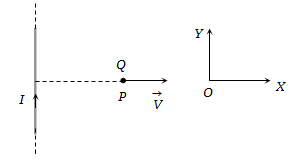1. Opposite to OX                            2. Along OX

3. Opposite to OY                             4. Along OY

Concept Questions :-

Lorentz force